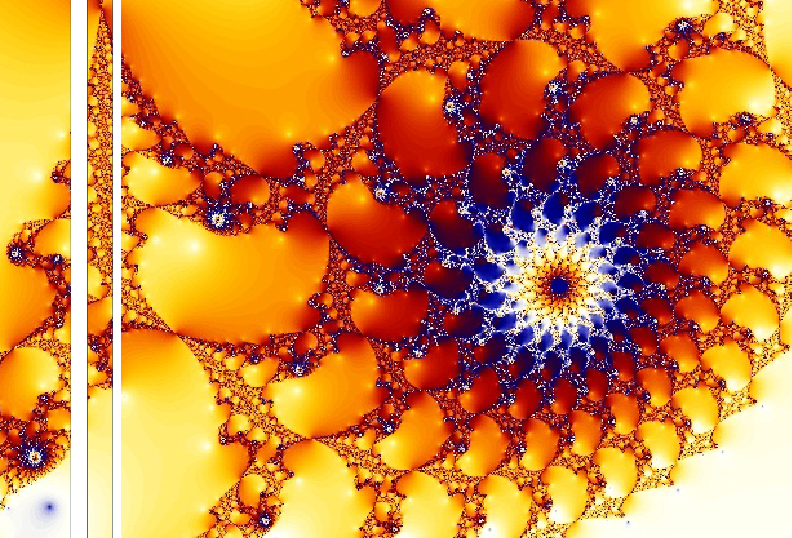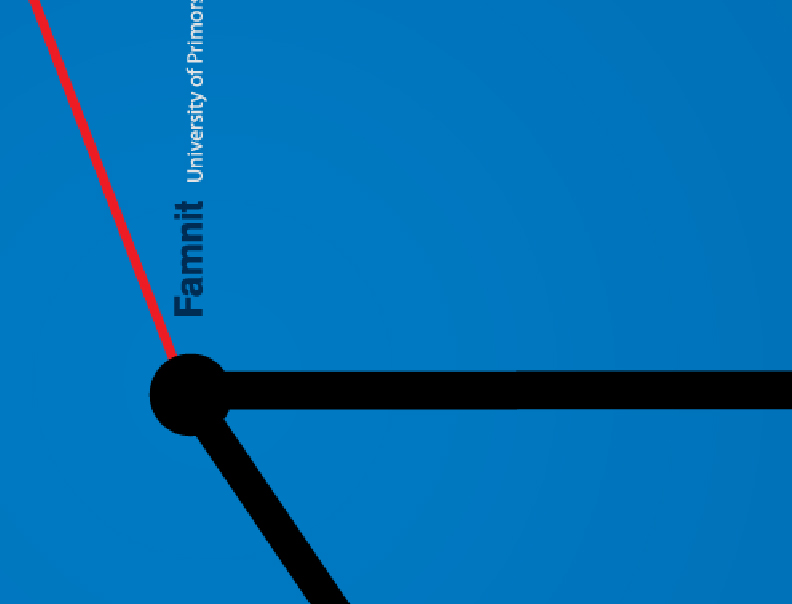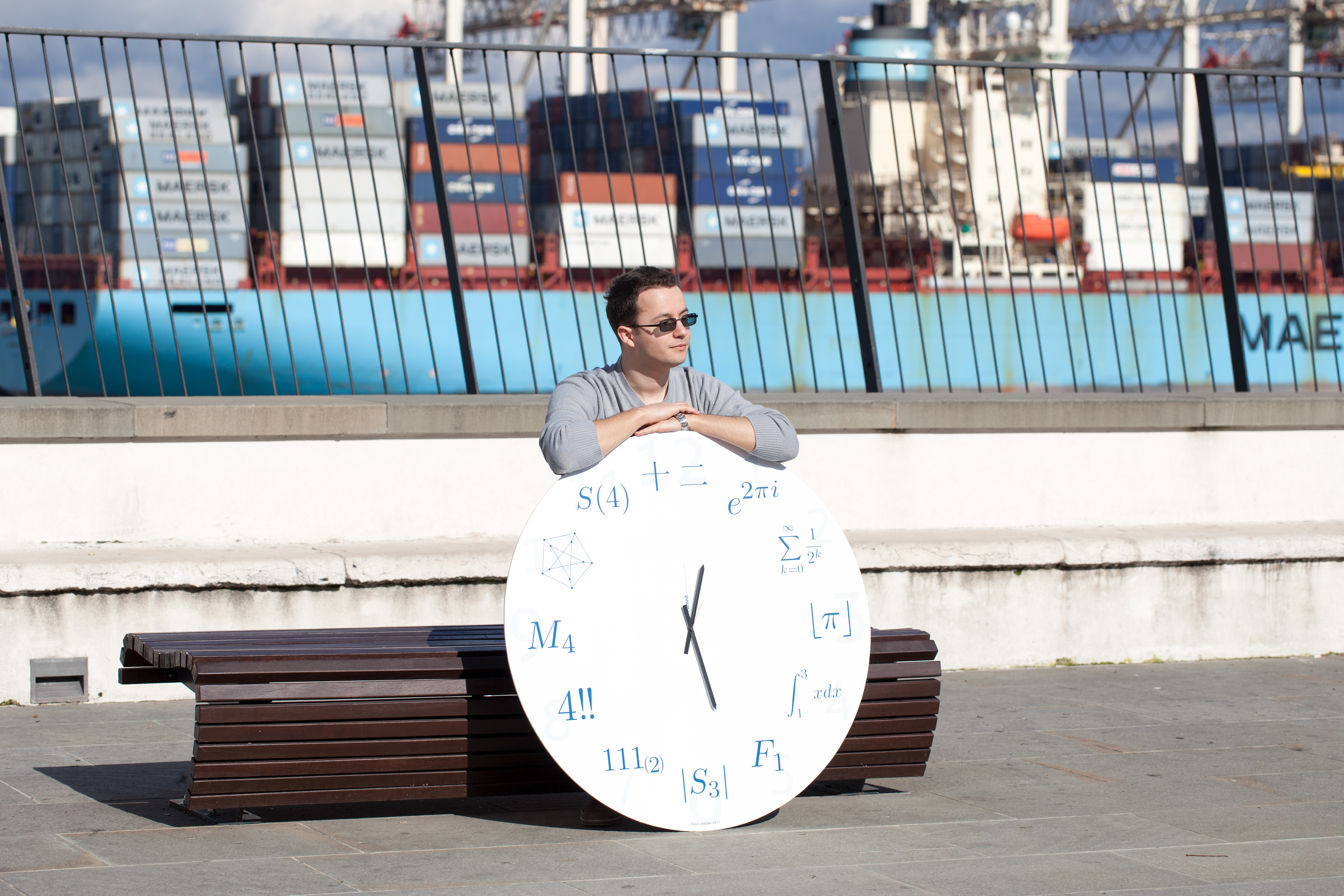Univerza na Primorskem Fakulteta za matematiko, naravoslovje in informacijske tehnologijeSI | EN# Raziskovalni matematični seminar - Arhiv

 2020 2019 2018 2017 2016 2015 2014 2013 2012 2011 2010
 1 2 3 4 5 6 7 8 9 10 11 12
28.03.2011. ob 10:00 Seminarska soba v Galebu
Predavatelj: dr.Martin Milanič

Title: Towards a combinatorial characterization of equistable graphs - partial results on a conjecture of Orlin

Abstract: Equistable graphs are graphs for which there exists a linear functional which characterizes the maximal stable sets of the graph. (A stable set is a set of pairwise non-adjacent vertices.) A conjecture due to Jim Orlin states that every equistable graph is a general partition graph (i.e., the intersection graph of a set system over a finite set S such that the maximal stable sets correspond precisely to the partitions of S using only sets from the family).

Orlin's conjecture holds within the class of chordal graphs; if true in general, it would provide a combinatorial characterization of equistable graphs. In this talk, we will explain the known connections between general partition graphs, equistable graphs, and triangle graphs, and show some partial results on Orlin's conjecture. In particular, we will present a complete characterization of equistable graphs decomposable with respect to the Cartesian or the tensor product.

21.03.2011. ob 10:00 Seminarska soba v Galebu
Predavatelj: mag. Boštjan Frelih

Title: Classification of cubic symmetric fivecirculants

Abstract: A fivecirculant is a graph admitting an automorphism having five cycles of equal length in its cycle decomposition. A graph is said to be symmetric if its automorphism group acts transitively on the set of its arcs. In this talk, we give a classification of cubic symmetric fivecirculants.

14.03.2011. ob 10:00 Seminarska soba v Galebu
Predavatelj: dr. Vito Vitrih

Title: High order parametric polynomial approximation of conic sections

Abstract: In this talk a particular shape preserving parametric polynomial approximation of conic sections will be presented. An ellipse and a hyperbola can be represented in a parametric form using e.g. trigonometric and hyperbolic functions. But, in contrast to a parabola, they do not have a parametric polynomial parameterization. In many applications a parametric polynomial approximation of conic sections is needed and it is important to derive accurate polynomial approximants. We will derive polynomial approximants with the highest possible approximation order in a closed form.